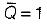Courses

# Sequential Logic Circuits - 2

## 15 Questions MCQ Test Topicwise Question Bank for Electrical Engineering | Sequential Logic Circuits - 2

Description
This mock test of Sequential Logic Circuits - 2 for Electrical Engineering (EE) helps you for every Electrical Engineering (EE) entrance exam. This contains 15 Multiple Choice Questions for Electrical Engineering (EE) Sequential Logic Circuits - 2 (mcq) to study with solutions a complete question bank. The solved questions answers in this Sequential Logic Circuits - 2 quiz give you a good mix of easy questions and tough questions. Electrical Engineering (EE) students definitely take this Sequential Logic Circuits - 2 exercise for a better result in the exam. You can find other Sequential Logic Circuits - 2 extra questions, long questions & short questions for Electrical Engineering (EE) on EduRev as well by searching above.
QUESTION: 1

### Assertion (A): The indeterminate condition of the J-K flip-flop is permitted in S-R flip-flop. Reason (R): A J-Kfiip-flop has a characteristic similar to that of an S-R flip-flop.

Solution:

The truth table for S-ffand J-Kflip-flop are shown below.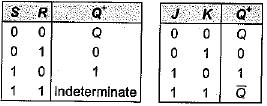It is clear from the above truth table that when S = R = 1, the output of S-R flip-flop is indeterminate. However, when J = K = 1, the output of J-Kflip-flop is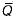(not indeterminate). Therefore, the indeterminate condition of the S-R flip-flop is permitted in a J-Kflip-flop.
A J-Kflip-flop has a characteristic similar to that of an S-R flip-flop which is evident from the above truth tables.
Hence, assertion is false but reason is true.

QUESTION: 2

### The J-K master-slave flip-flops in figure shown below has its J and K inputs tied to + VCC and a series of pulses is applied to its CLK input.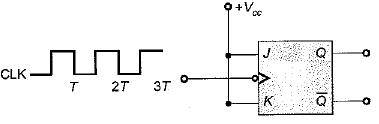The waveform at G is given by

Solution:

Let the flip-flop is initially reset.
The truth table for aiven circuit is shown below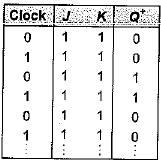Thus, the waveform for Q will be as shown below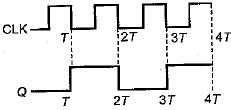QUESTION: 3

### For the J-Kflip-flop shown below to function as a divide-by-2 element, the J and K inputs should be equal to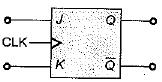Solution:

If J = K = 1, then the truth table will be as show below (Initially flip-flop is.reset).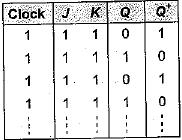Thus, the given J-Kflip-flop will work as a divide- by-2 elemen

QUESTION: 4

Match List-l with List-II and select the correct answer using the codes given below the lists:
List-I
A. J-K flip-flop
B. D flip-flop
C. Master-slave flip-flop
D. T flip-flop
List-lI
1. Transparent latch
2. Most versatile and widely used of all the flip flops
3. Not widely available as commercial items
4. Pulse triggered flip-flop
Codes: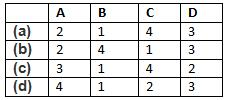Solution:

• The J-K flip-flop is the most versatile and most widely used of all the flip-flops.
• Transparent latch is associated with D-flip flop since the clocked D latch is called a transparent D latch, because its output follows the input when the clock is HIGH.
• Master-slave flip-flops are called pulse- triggered flip-flops, because the length of time required for its output to change state equals the width of one (clock) pulse.
• T flip -flop is not widely available as commercial items.

QUESTION: 5

Assertion (A): An unclocked flip-flop is called a latch.​
Reason (R): A latch is constructed using two cross-coupled NAND gates or NOR gates.

Solution:

An unclocked flip-flop is called a latch, because the output of the flip-flop latches on to ‘1’ or ‘0' immediately after the input is applied. Hence, assertion is a correct statement. Reason is also a true statement but not the correct explanation of assertion.

QUESTION: 6

Consider the following statements associated with flip-flops;
1. Any one type of flip-flop can be converted to any other type by providing a suitable combinational circuit.
2. The inputs to a master-slave flip-flop must change when the clock is HIGH.
3. In a master-slave flip-flop, master is level triggered while slave is edge triggered.
4.  Data lock-out flip-flops are nothing but master-slave flip-flops.

Q. Which of the statements given above is/are not correct?

Solution:

• Statement-1 is correct.
• The inputs to a master-slave flip-flops must not change when the clock is HIGH. If they change, the flip-flop gives an output corresponding to the last combination of inputs present before the dock terminals. Hence, statement-2 is not correct.
• In a master-slave flip-flop, master is edge-triggered while slave is level triggered. Thus, statements-3 is not correct.
• Data lock-out flip-flops are nothing but master-slave flip-flops in which the master is an edge triggered flip-flop. Thus, statement-4 is correct.

QUESTION: 7

For the flip-flop shown below, there is one clock pulse for each bit time.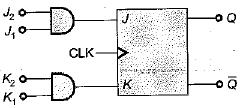If the following serial data are applied to the flip-flop, then the resulting decimal value of the serial data that appears on the Q output will be (assume that, Q is initially ‘0’)
J1 = 01101101 ; J2 =10011011
K1 = 01101001 ; K2 = 11011011

Solution:

Q = 0 (Initially)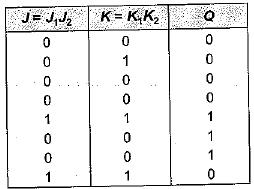Thus, serial output data at Q
= 00001110 = 14 (Decimal value)

QUESTION: 8

For a flip-flop formed from two NOR gates as shown in figure the unusable state corresponds to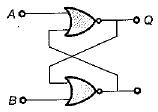Solution:

Given flip-flop represents S-R latch using NOR gates.
When A = 1, B = 1 we will get invalid or unusable state.

QUESTION: 9

The digital circuit as shown below represents to which one of the following?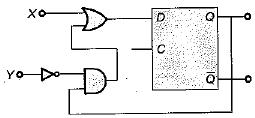Solution:

Here,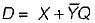If X = S and Y = R then, the given circuit circuit represents S-R flip-flop using D flip-flop

QUESTION: 10

If tp is the pulse width, Δt is the propagation delay, T is period of pulse train then which of the following condition can avoid the race around condition?

Solution:

Race around condition occurs in J-K flip-flop when J = K = 1. This condition can be avoided if the propagation delay of flip-flop is more than pulse-width of the clock but less than the clock.
i.e. 2tp < Δt < T

QUESTION: 11

Match List-I (Logic Circuit) with List-ll (Circuit Realization) and select the correct answer using the codes given below the lists:
List-I
A. J-K flip-flop
B. T flip-flop
C. D fiip-flop
List-ll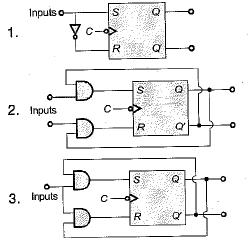Codes: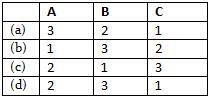Solution:

For conversion of S-R flip-flop to
A. J-K flip flop:
S = JQ' and R = KQ
B. T Flip-flop:
S = TQ' and = TQ
C. D flip-flop: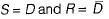QUESTION: 12

The characteristic equation of a T flip-flop is given by

Solution:

For a T flip-flop, Qn+1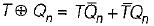QUESTION: 13

In a J-Kflip-flop we have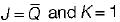(shown in figure). Assuming the flip-flop was initially cleared and then clocked for 6-pulses, the sequence at the Q output will be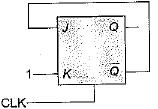Solution:

The truth table will be as shown below.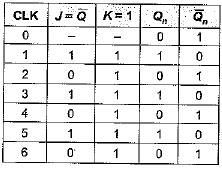Thus, output Q for 6 clock pulse = 101010.

QUESTION: 14

The output of a J-Kflip-flop Qn is ‘0’. The state of the flip-flop changes when a clock pulse is applied. The possible combination of Jn and Kn inputs could be (‘X' denote don’t care)

Solution:

The truth table of J-K flip-flop is shown below.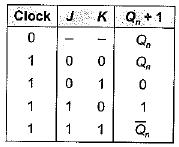Given, Qn = 0 initially,
When clock pulse is applied, the state of FF changes to ‘1’ i.e. Qn+ 1 = 1, which is possible,
if, ' J = 1, K = 0 ...(i)
or, Jn = 1 , Kn = ‘X’ ...(ii)
Thus, Jn = 1 and Kn = ‘X (don't care) satisfy the above two conditions.

QUESTION: 15

When a flip-flop is reset, its output will be

Solution:

A FF is set when Q = 1,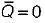A FF is reset when  Q = 0 ;